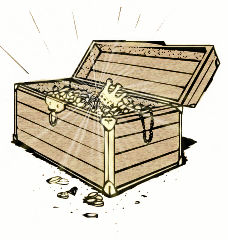# All That Glitters Is Gold

Probability Level 3There are three boxes:
1. a box containing 2 gold coins,
2. a box containing 2 silver coins,
3. a box containing 1 gold coin and 1 silver coin.

After choosing a box at random and picking out one coin, you withdrew a gold coin. If the chances that the other coin in the box is gold can be represented as $\frac {a}{b}$ where $a$ and $b$ are co-prime, find the value of $a + b$.

×

Problem Loading...

Note Loading...

Set Loading...